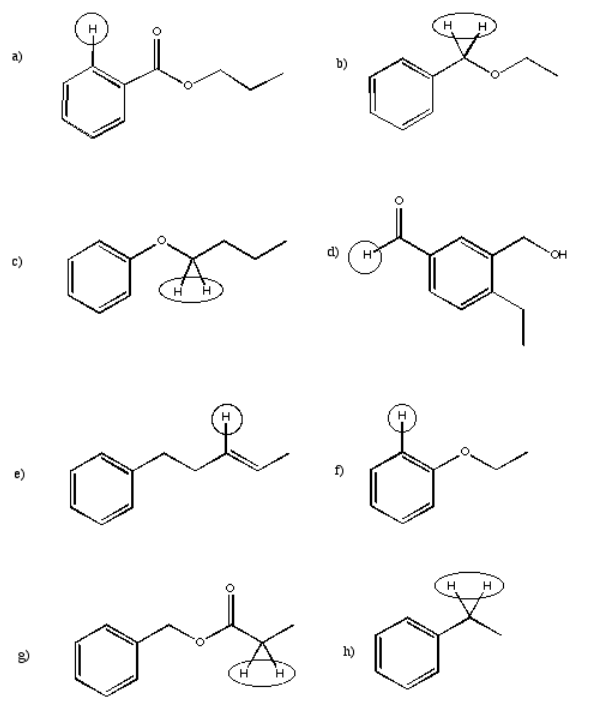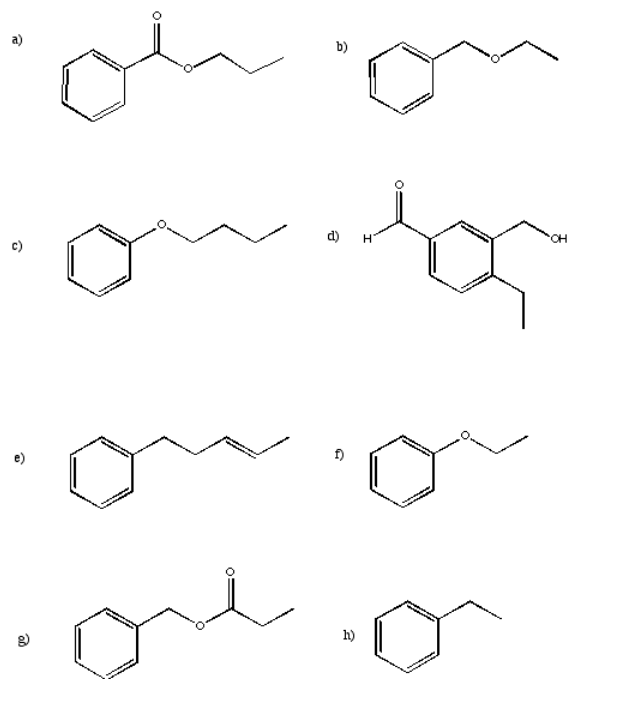Skip to main content

# 4.16: More Practice

Exercise $$\PageIndex{1}$$

For each of the following structures, indicate how many peaks would be found in the 13C spectrum.Exercise $$\PageIndex{2}$$

Sketch the expected 13C spectrum for each of the structures in the previous question.

Exercise $$\PageIndex{3}$$

Suggest possible assignments for peaks found at the following positions in the 13C NMR spectrum.

a) 63 ppm b) 114 ppm c) 205 ppm d) 35 ppm e) 165 ppm f) 175 pp

Exercise $$\PageIndex{4}$$

Suggest the approximate chemical shift for the circled carbons in the following partial structures.Exercise $$\PageIndex{5}$$

Explain why, in the following cases, chemical shift is slightly different from the normal range described.

a) chlorofom (CHCl3): H on sp3 carbon; normally 0-5 but here at 7.27 ppm.

b) vinyl ether (CH2=CHOCH=CH2): H on sp2 carbon normally 5-7 but here at 4.5 ppm.

c) nitrobenzene (C6H5NO2): H on sp2, aromatic carbon normally 7-8 but here 8.5 ppm

Exercise $$\PageIndex{6}$$

Suggest possible assignments for peaks found at the following positions in the 1H NMR spectrum.

a) 7.4 ppm b) 12.1 ppm c) 3.6 ppm d) 10.1 ppm e) 8.2 ppm f) 2.1 ppm g) 5.8 ppm

Exercise $$\PageIndex{7}$$

Suggest the approximate chemical shift for the circled protons in the following partial structures.Exercise $$\PageIndex{8}$$

Suggest the arrangement of neighbouring hydrogens for the following peaks in the 1H NMR spectrum and draw a partial structure.Exercise $$\PageIndex{9}$$

Sketch peak shapes for the circled protons in the following partial structures.Exercise $$\PageIndex{10}$$

Describe the different coupling patterns in the aromatic region of the 1H NMR spectra of the following isomers.Exercise $$\PageIndex{11}$$

Assign the relative number of protons at each position based on the integral lines shown.Exercise $$\PageIndex{12}$$

Suggest partial structures for the following data, given in string form.

a) 8.05 ppm (doublet, 2H) b) 3.25 ppm (septet, 1H) c) 2.65 ppm (nonet, 1H)

d) 6.55 ppm (broad singlet, 1H) e) 0.94 ppm (triplet, 3H) f) 2.33 ppm (broad singlet, 2H)

g) 8.65 ppm (singlet, 1H) h) 2.05 ppm (quartet, 2H) i) 6.21 ppm (doublet of doublets, 1H)

Exercise $$\PageIndex{13}$$

Suggest complete structures from the following sets of partial structures.Exercise $$\PageIndex{14}$$

Show complete analysis of the following spectral data and propose a structure in each case.

a)b)c)d)Exercise $$\PageIndex{15}$$

Sketch the expected 1H spectrum for each of the following structures.Exercise $$\PageIndex{16}$$

Draw what the NMR spectrum of ethylbenzene, CH3CH2C6H5, would look like if it was contaminated with an equal amount of tert-butyl methyl ether, (CH3)3COCH

Exercise $$\PageIndex{17}$$

The following NMR spectrum, of the aldehyde shown, is contaminated with another isomer.1. Identify the peaks corresponding to each isomer.
2. Identify which peaks correspond to which proton in each isomer.
3. Identify the ratio of isomers in the sample.